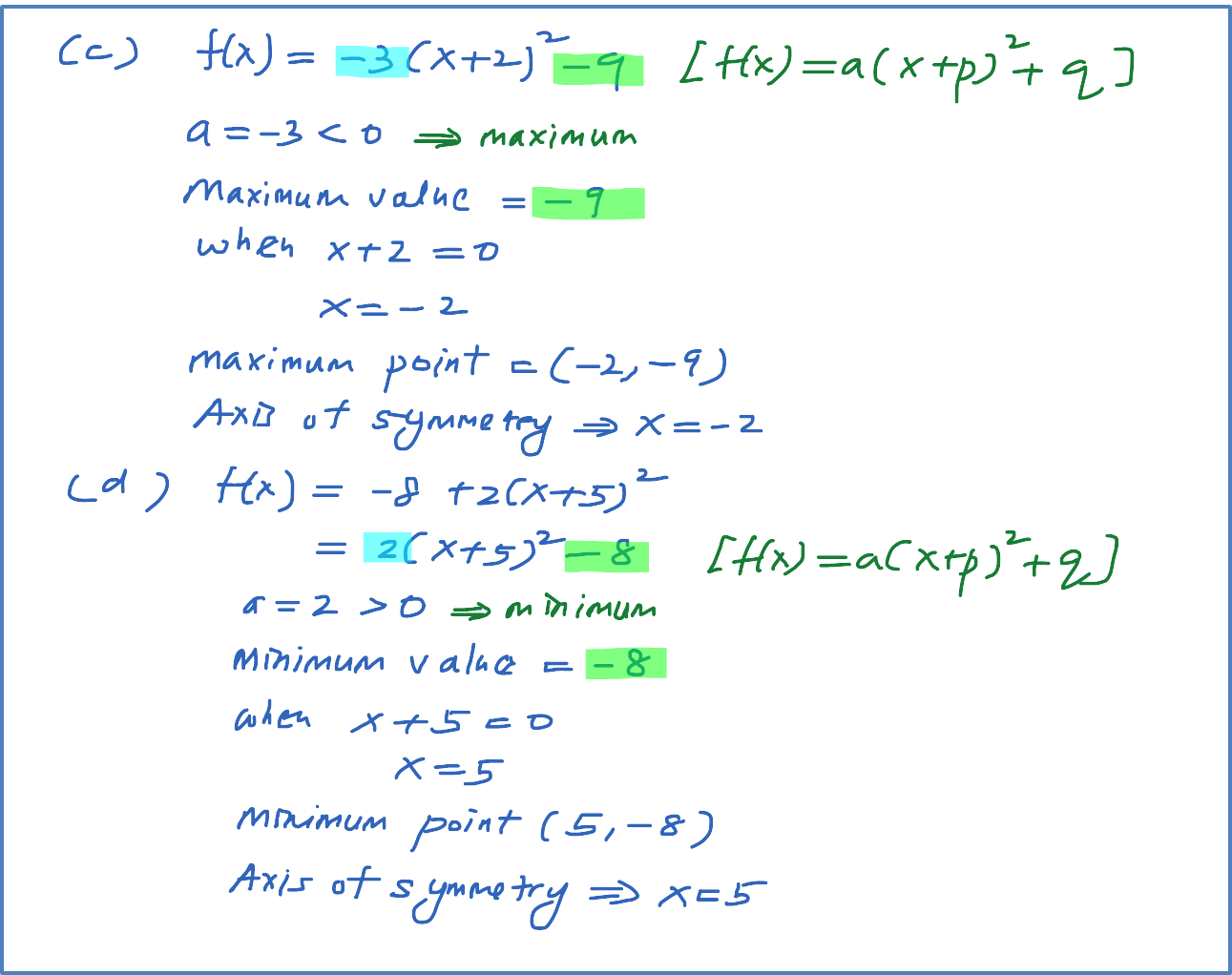# 3.3.1 Example 1 : Finding the maximum/minimum and axis of symmetry of a quadratic function

Example 1
State the maximum or minimum value of   for each of the following quadratic function and state the value of x at which the function is maximum or minimum.  Find the maximum or minimum point and finally state axis of symmetry for each case.

(a) $f\left(x\right)=-2{\left(x-3\right)}^{2}+4$
(b) $f\left(x\right)=3{\left(x-4\right)}^{2}+10$
(c) $f\left(x\right)=-3{\left(x+2\right)}^{2}-9$
(d) $f\left(x\right)=-8+2{\left(x+5\right)}^{2}$Correction for part (d) of the question,
when x + 5 = 0, x = -5
minimum point is (-5, -8)
Axis of symmetry, x = -5